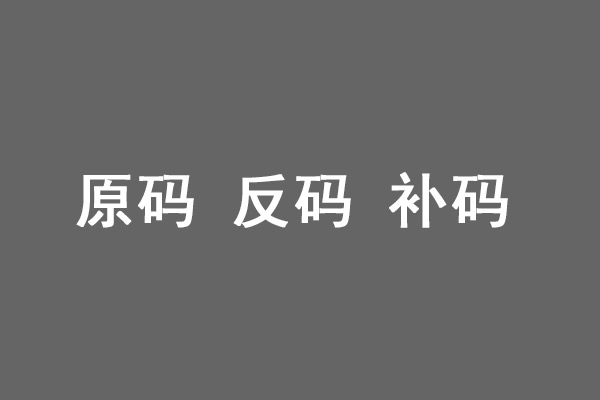﻿ 为什么要用原码，反码和补码？原码，反码，补码介绍

# 为什么要用原码，反码和补码？原码，反码，补码介绍

1、机器数

2、真值

0000 0001的真值 = +000 0001 = +1

1000 0001的真值 = –000 0001 = –11. 原码

[+1]（原码） = 0000 0001

[-1]（原码） = 1000 0001

[1111 1111 , 0111 1111]

[-127 , 127]

2. 反码

[+1] = （原码）= （反码）

[-1] = （原码）= （反码）

3. 补码

[+1] = （原码） = （反码） = （补码）

[-1] = （原码） = （反码） = （补码）

[+1] = （原码） = （反码） = （补码）

[-1] = （原码） = （反码） = （补码）

1 - 1 = 1 + (-1) = 原 + 原 = 原 = -2

1 - 1 = 1 + (-1) = [0000 0001]原 + [1000 0001]原= [0000 0001]反 + [1111 1110]反 = [1111 1111]反 = [1000 0000]原 = -0

1-1 = 1 + (-1) = [0000 0001]原 + [1000 0001]原 = [0000 0001]补 + [1111 1111]补 = [0000 0000]补=[0000 0000]原

(-1) + (-127) = [1000 0001]原 + [1111 1111]原 = [1111 1111]补 + [1000 0001]补 = [1000 0000]补

-1-127的结果应该是-128，在用补码运算的结果中，[1000 0000]补 就是-128。但是注意因为实际上是使用以前的-0的补码来表示-128，所以-128并没有原码和反码表示 (对-128的补码表示[1000 0000]补算出来的原码是[0000 0000]原，这是不正确的)。